# JP5538203B2 - Power fluctuation detection device and power fluctuation detection method - Google Patents

## Info

Publication number
JP5538203B2
JP5538203B2 JP2010291101A JP2010291101A JP5538203B2 JP 5538203 B2 JP5538203 B2 JP 5538203B2 JP 2010291101 A JP2010291101 A JP 2010291101A JP 2010291101 A JP2010291101 A JP 2010291101A JP 5538203 B2 JP5538203 B2 JP 5538203B2
Authority
JP
Japan
Prior art keywords
gauge
amplitude
voltage
effective value
vibration frequency
Prior art date
Legal status (The legal status is an assumption and is not a legal conclusion. Google has not performed a legal analysis and makes no representation as to the accuracy of the status listed.)
Active
Application number
JP2010291101A
Other languages
Japanese (ja)
Other versions
JP2012139067A (en
Inventor

Original Assignee

Priority date (The priority date is an assumption and is not a legal conclusion. Google has not performed a legal analysis and makes no representation as to the accuracy of the date listed.)
Filing date
Publication date
Application filed by 三菱電機株式会社 filed Critical 三菱電機株式会社
Priority to JP2010291101A priority Critical patent/JP5538203B2/en
Publication of JP2012139067A publication Critical patent/JP2012139067A/en
Application granted granted Critical
Publication of JP5538203B2 publication Critical patent/JP5538203B2/en
Application status is Active legal-status Critical
Anticipated expiration legal-status Critical

## Images

•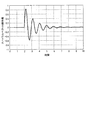•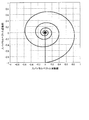•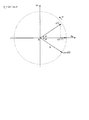•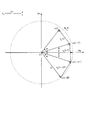•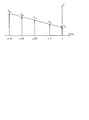•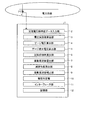•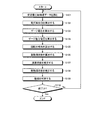•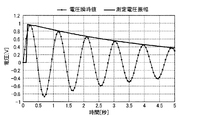•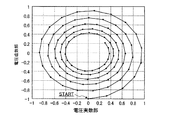•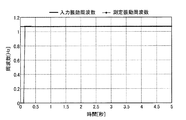•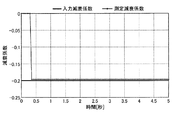•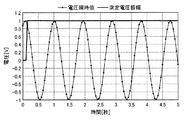•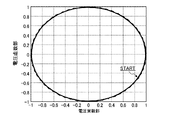•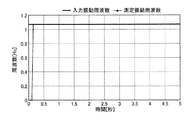•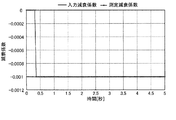•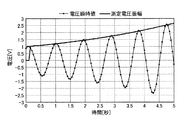•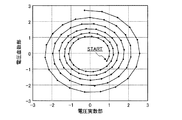•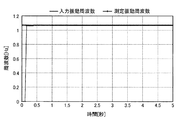•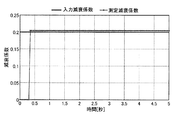•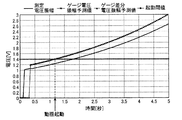•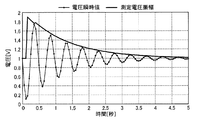•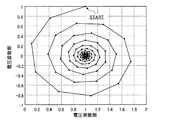•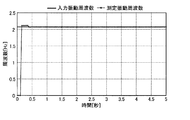•## Description

The present invention relates to a power fluctuation detection device and a power fluctuation detection method for detecting fluctuations in a power system.

In an electric power system, there are various parameters such as the effective power of a transmission line, the phase angle of a generator, the effective voltage value of a substation, and the actual frequency at the substation / power plant. In order to operate the power system stably, it is required to always maintain these parameters within a certain range.

Conventionally, when the rate of change of the power waveform changes from positive to negative after detecting and storing the sign change of the rate of change of the power oscillation waveform and the bottom of the power oscillation waveform, it is recognized as the peak of the power oscillation waveform and vice versa. When the rate of change of the power waveform changes from negative to positive after detecting and storing the peak of the power oscillation waveform, it is recognized that it is the bottom of the power oscillation waveform. Thus, there has been disclosed a power fluctuation detection device that can respond correctly (for example, Patent Document 1).

The following Patent Documents 2 and 3 are prior inventions by the inventor of the present application and will be described later.

JP 59-80114 A JP 2007-60870 A International Publication No. 2008/120282

However, the technique disclosed in Patent Document 1 determines whether the rate of change of the power waveform is positive or negative. This also means that the fluctuation of the power system cannot be detected with high accuracy in a short time. In other words, the power fluctuation detection device of Patent Document 1 has a problem that it cannot detect the fluctuation of the power system at high speed and with high accuracy.

The present invention has been made in view of the above, and an object of the present invention is to provide a power fluctuation detection device and a power fluctuation detection method capable of detecting a fluctuation of a power system at high speed and with high accuracy.

In order to solve the above-described problems and achieve the object, the present invention provides a power fluctuation detection device that detects a fluctuation of an electric power system based on an effective value of an AC electric quantity measured in the electric power system, The gauge amplitude, which is a normalized value of the amplitude value obtained by integrating the instantaneous value data of at least three consecutive points obtained by sampling the effective value of the AC electric quantity at a sampling frequency that is at least twice the main vibration frequency at the effective value, Gauge amplitude calculation unit to calculate, and two adjacent instantaneous values in at least four consecutive instantaneous value data including three instantaneous value data sampled at the sampling frequency and used for calculating the gauge amplitude Gauge differential amplitude that calculates the gauge differential amplitude, which is the normalized value of the value obtained by integrating the differential instantaneous value data of the three points representing the tip-to-tip distance between the data A rotation phase angle calculation unit that calculates a rotation phase angle in one sampling period using the gauge amplitude and the gauge differential amplitude, and calculates the main vibration frequency using the rotation phase angle and the sampling frequency. A vibration frequency calculating unit that applies a regression analysis method to the time series data of the gauge amplitude, calculates a damping coefficient corresponding to the main vibration frequency of the gauge amplitude, and the gauge amplitude and the gauge differential amplitude. A fluctuation determination unit that determines whether or not the fluctuation of the power system tends to diverge based on the predicted values of the gauge amplitude and the gauge differential amplitude after a predetermined time calculated using To do.

According to the present invention, there is an effect that it is possible to provide a power fluctuation detection device and a power fluctuation detection method for detecting fluctuations of a power system at high speed and with high accuracy.

FIG. 1 is a diagram showing spiral vector instantaneous values in the time domain. FIG. 2 is a diagram showing spiral vectors on the complex plane. FIG. 3 is a diagram for explaining a group of gauge voltages on the complex plane. FIG. 4 is a diagram for explaining a gauge differential voltage group on a complex plane. FIG. 5 is a diagram showing the transition of the gauge voltage when the gauge voltage can be linearly approximated. FIG. 6 is a diagram illustrating a functional configuration of the power fluctuation detection device according to the present embodiment. FIG. 7 is a flowchart showing the flow of processing in the power fluctuation detection device. FIG. 8 is a diagram illustrating a waveform of an instantaneous voltage value and a measured voltage amplitude in case 1. FIG. 9 is a diagram illustrating a voltage spiral vector on the complex plane in case 1. In FIG. FIG. 10 is a diagram illustrating the input vibration frequency and the measurement vibration frequency in Case 1. FIG. 11 is a diagram illustrating an input attenuation coefficient and a measured attenuation coefficient in Case 1. FIG. 12 is a diagram illustrating a waveform of an instantaneous voltage value and a measured voltage amplitude in case 2. FIG. 13 is a diagram illustrating voltage spiral vectors on the complex plane in Case 2. In FIG. FIG. 14 is a diagram illustrating the input vibration frequency and the measurement vibration frequency in Case 2. FIG. 15 is a diagram illustrating an input attenuation coefficient and a measured attenuation coefficient in Case 2. FIG. 16 is a diagram showing a waveform of a voltage instantaneous value and a measured voltage amplitude in case 3. FIG. 17 is a diagram illustrating a voltage spiral vector on the complex plane in case 3. In FIG. FIG. 18 is a diagram illustrating the input vibration frequency and the measurement vibration frequency in Case 3. FIG. 19 is a diagram illustrating an input attenuation coefficient and a measured attenuation coefficient in Case 3. FIG. 20 is a diagram for explaining the motion determination result in case 3. FIG. FIG. 21 is a diagram showing the waveform of the voltage instantaneous value and the measured voltage amplitude in case 4. FIG. 22 is a diagram illustrating a voltage spiral vector on the complex plane in Case 4. In FIG. FIG. 23 is a diagram illustrating an input vibration frequency and a measurement vibration frequency in Case 4. FIG. 24 is a diagram illustrating an input attenuation coefficient and a measured attenuation coefficient in Case 4.

Hereinafter, a power fluctuation detection apparatus and a power fluctuation detection method according to embodiments of the present invention will be described with reference to the accompanying drawings. In addition, this invention is not limited by embodiment shown below.

(Meaning of terms)
In describing the power fluctuation detection apparatus and the power fluctuation detection method according to the present embodiment, first, terms used in this specification will be described.

System frequency: Basically, it means the rated frequency in the power system, and there are two types, 50 Hz and 60 Hz. It should be noted that the actual system frequency slightly changes in the vicinity of the rated frequency even when the power system is stable.
Spiral vector: A rotation vector that decreases or expands while rotating counterclockwise on the complex plane. In the present invention, the spiral vector can be actually measured by separately measuring the vibration frequency and the damping coefficient of the spiral vector.
Circle vector: When the damping coefficient is zero, the spiral vector is a circle vector. Note that the state in which the spiral vector is represented by a circle vector corresponds to a steady AC state.
-Circle vector symmetry: Refers to the property that the symmetry group of a circle vector has complete symmetry. In the present invention, it is proposed that the system frequency is obtained by utilizing the circular vector symmetry assuming that the magnitude of the vector amplitude is constant within a certain time range.
Spiral vector symmetry: Refers to the property that the symmetry group of spiral vectors has a certain symmetry. It is to be noted that the present invention proposes to obtain the oscillation frequency of the oscillating vibration using the spiral vector symmetry, assuming that the magnitude of the amplitude of the measurement object slightly changes within a certain time range.
・ Vibration frequency: An amount that represents the rate of change (vibration) in AC electricity such as power, phase angle, voltage effective value, current effective value, system frequency, etc. ("vibration frequency", "fluctuating frequency", etc.) In other words). That is, it should be noted that this vibration frequency is a concept different from the system frequency. This vibration frequency changes according to the system characteristics. For example, even when the power system does not become unstable, it is said that the vibration frequency can change within a range of several Hz (for example, 0.5 to 4 Hz).
Gauge voltage group: a symmetric group composed of three voltage rotation vectors continuous in time series. The same symmetrical group concept can be defined for currents other than voltage and power (active power and reactive power).
Gauge voltage: A voltage invariant calculated by the gauge voltage group.
Gauge differential voltage group: A symmetric group composed of three differential voltage rotation vectors that are continuous in time series.
Gauge differential voltage: a differential voltage invariant calculated by a gauge differential voltage group.

In the specification of the present application, a calculation formula using an example of a gauge voltage / gauge differential voltage based on an AC electric quantity called a voltage will be described, but the active power (cage effective power / cage differential active power), generator phase angle (cage) Conversion to various calculation formulas such as generator phase angle / cage differential generator phase angle), system frequency (cage frequency / cage differential frequency), inter-bus phase difference (phase difference between one bus and another) Can do. Hereinafter, these concepts are collectively referred to as gauge amplitude and gauge differential amplitude, respectively. In addition, each component in the power fluctuation detection device described below and each step in the power fluctuation detection processing method are also read as gauge amplitude or gauge difference amplitude.

(Embodiment)
Next, the concept (algorithm) of the motion detection technique forming the gist of the present embodiment will be described.

FIG. 1 is a diagram showing spiral vector instantaneous values in the time domain. The spiral vector instantaneous value shown in FIG. 1 can be expressed as follows.

In the above equation (1), P S is an initial amplitude value, λ is an attenuation coefficient, ω is a vibration angular frequency, f is a vibration frequency, and φ is an initial phase.

FIG. 2 is a diagram showing spiral vectors on the complex plane. The spiral vector shown in FIG. 2 can be expressed by the following equation.

Further, the real part (P re ) and the imaginary part (P im ) of the spiral vector can be expressed as follows.

As can be understood from the above equations, if the attenuation coefficient λ and the vibration frequency f of the spiral vector can be measured, the fluctuation waveform of the spiral vector can be determined. In particular, when the damping coefficient λ is greater than zero, it can be determined that the fluctuations diverge, and appropriate system control can be executed. The system control includes a generator shutoff control, a system separation control, and the like, and stable operation of the power system can be maintained through these controls.

By the way, an actual oscillation waveform has a plurality of damping coefficients respectively corresponding to a plurality of vibration frequencies. However, within a certain time width, a fluctuation waveform is generated with one main vibration frequency (main vibration frequency, hereinafter simply referred to as “vibration frequency”) corresponding to the selected sampling frequency and a damping coefficient corresponding to the vibration frequency. It is possible to simulate approximately. However, it is important to select a sampling frequency that is suitable for the subject of study. For example, since the oscillation period differs between the examination of the incoming current of a transmission line lightning and the long-period power fluctuation of the power system, it is necessary to appropriately select each sampling frequency.

Next, a method for obtaining the attenuation coefficient and vibration frequency of the spiral vector will be described with reference to FIGS. In addition, although it was mentioned above, the calculation formula which uses a gauge voltage / gauge differential voltage as an example is demonstrated here.

FIG. 3 is a diagram for explaining a group of gauge voltages on the complex plane. In FIG. 3, on the complex plane, the current voltage rotation vector v (t) and the voltage rotation vector v (t−) at the time before the current sampling period T (a time corresponding to the sampling frequency 1 step width) from the current time point. T), a voltage rotation vector v (t−2T) at a time point two sampling periods (2T) before the current time point is shown. In the following description, v (t) is an instantaneous value of the voltage effective value (that is, a value when time variation of the voltage effective value is observed). For example, “v” expressed without parentheses represents the real part of the instantaneous value of the voltage effective value. Further, for example, “V” expressed in capital letters of the alphabet represents an effective value or an amplitude value of voltage effective value (hereinafter simply referred to as “voltage amplitude”).

Now, consider these three voltage rotation vectors. First, these three voltage rotation vectors are rotation vectors that rotate counterclockwise on the complex plane at the same rotation speed, and are expressed as follows using the sampling period T.

In the above equation (4), V is the voltage amplitude described above. Further, ω is a rotational angular velocity (also a vibration angular frequency) and is expressed by the following equation.

In the above equation (5), f is the vibration frequency. Also, the sampling one period T in the equation (4) is expressed by the following equation.

In the above equation (6), f S is a sampling frequency. Further, α shown in the equation (4) is a rotation phase angle that means an angle at which the voltage vector is rotated on the complex plane in the time of one sampling period T.

In FIG. 3, the voltage vectors (v (t), v (t−2T)) on both sides have approximate symmetry with respect to the intermediate voltage vector (v (t−T)). Note that approximate means that there is a slight difference in the amplitude value between the voltage vector v (t) and the voltage vector v (t−2T). This is not a problem when considering symmetry. These three voltage rotation vectors form one voltage rotation vector group that rotates counterclockwise on the complex plane at the same rotation speed, and a normalized value (gauge voltage) as described later is obtained. Defined. Due to these properties, a group of these three voltage rotation vectors is defined as a gauge voltage group.

Next, a calculation formula of the gauge voltage V g which is an amplitude value (normalized amplitude value) of the gauge voltage group will be described. First, the equation for calculating the gauge voltage V g is defined as follows:

In the above equation (7), v 2 is the real part of the second voltage rotation vector in the gauge voltage group, v 1 is the real part of the first voltage rotation vector in the gauge voltage group, and v 3 is the third part in the gauge voltage group. The real part of the voltage rotation vector of

In the above equation (8), the symbol “Re” indicates the real part of the complex vector component. Here, if the expression (8) is substituted into the right side of the expression (7), it is expanded as the following expression.

That is, the gauge voltage V g is expressed by the following equation.

As represented by the above equation (10), the gauge voltage V g is represented by the product of the term of the voltage amplitude V, the term of the sine function of the rotational phase angle α, and the DC attenuation term. Since the vibration frequency f and the rotation phase angle α have a one-to-one correspondence, the vibration frequency f and the attenuation coefficient λ included in the DC attenuation term can be separated by the above-described equation modification.

FIG. 4 is a diagram for explaining a gauge differential voltage group on a complex plane. In FIG. 4, on the complex plane, the current voltage rotation vector v (t), and the voltage rotation vector v (t−) at the time before the current sampling period T (a time corresponding to the sampling frequency 1 step width) before the current time. T), a voltage rotation vector v (t-2T) at a time point 2 sampling (2T) before the current time point, and a voltage rotation vector v (t-3T) at a time point 3 sampling (3T) time point before the current time. Yes.

Further, in FIG. 4, v 2 (t), which is a voltage difference vector between v (t) and v (t−T), and a voltage difference vector between v (t−T) and v (t−2T). The voltage difference vector v 2 (t−2T) between v 2 (t−T), v (t−2T) and v (t−3T) is shown. These three voltage difference vectors are expressed by the following equation using the voltage amplitude V, the rotation angular velocity ω, and the rotation phase angle α, similarly to the three voltage rotation vectors described above.

Here, referring to FIG. 4, among the three voltage difference vectors, the voltage difference vectors (v 2 (t), v 2 (t−2T)) on both sides are equal to the intermediate voltage difference vector (v 2 (t− There is an approximate symmetry for T)). Note that approximate means that there is a slight difference in amplitude value between the voltage difference vector v 2 (t) and the voltage difference vector v 2 (t−2T). Since it is smaller than time, there is no problem in considering symmetry. These three voltage difference vectors form one voltage difference vector group that rotates counterclockwise on the complex plane at the same rotation speed, and one normalized value (gauge difference voltage as described later). ) Is defined. Due to these properties, a group of these three voltage difference vectors is defined as a gauge difference voltage group.

Next, a calculation formula of the gauge differential voltage V gd that is the amplitude value (normalized amplitude value) of the gauge differential voltage group will be described. First, the calculation formula of the gauge differential voltage V gd is defined as follows.

In the above equation (12), v 22 is the real part of the second voltage difference vector in the gauge difference voltage group, v 21 is the real part of the first voltage difference vector in the gauge difference voltage group, and v 23 is the gauge difference voltage group. Is the real part of the third voltage difference vector in FIG.

In the above equation (13), the symbol “Re” indicates the real part of the complex vector component. Here, if the equation (13) is substituted into the equation in the square root symbol on the right side of the equation (12), the following equation is developed.

In the development of the above formula, the following approximate formula is used.

Therefore, the gauge differential voltage V gd can be expressed by the following formula by substituting the formula (14) into the formula (12).

As expressed by the above equation (16), the gauge differential voltage V gd is similar to the gauge voltage V g in that the term of the voltage amplitude V, the term of the sine function of the rotational phase angle α, and the DC attenuation term The vibration frequency f and the attenuation coefficient λ included in the DC attenuation term can be separated.

Further, the following relational expression is obtained from the above expressions (10) and (16).

Therefore, the rotation phase angle α is expressed by the following equation using the gauge voltage V g and the gauge differential voltage V gd .

In general, since one period T of the sampling frequency is very small (for example, when the sampling frequency is 1000 Hz, one period T is 0.001 second), it may be considered that the following equation holds.

Therefore, the rotational phase angle α can be calculated using the following equation obtained by substituting the relationship of the equation (19) into the equation (18).

The vibration frequency f can be calculated using the following equation.

Furthermore, the vibration angular frequency ω can be calculated using the following equation.

Further, the equation for obtaining the voltage amplitude V by the equation (10) can be expressed as the following equation using the gauge voltage V g and the rotation phase angle α.

Moreover, the equation for obtaining the voltage amplitude V can also be expressed as the following equation using equation (16).

Here, the sine function value and cosine function value of the rotational phase half angle (α / 2) can be expressed by the following equation and the following equation by the equation (20).

Therefore, the voltage amplitude V can be calculated using the following equation by the above equations (23), (25), and (26).

Up to this point, the gauge voltage determined by the gauge voltage group based on the three voltage rotation vectors (3 sampling points) and the three voltage rotation vectors based on the two adjacent voltage rotation vectors among the four voltage rotation vectors (4 sampling points). Although each calculation formula of the gauge difference voltage obtained by the gauge difference voltage group based on the voltage difference vector has been shown, it is not limited to these sampling points in calculating the gauge voltage and the gauge difference voltage, but the number of sampling points It is also possible to increase. Therefore, hereinafter, a calculation formula when the number of sampling points is n is presented.

First, the calculation formula of the gauge voltage by (n−2) gauge voltage groups using n sampling points (n is 3 or more) voltage rotation vectors is as follows.

That is, the gauge voltage represented by the above equation (28) is a square integral calculation of effective value data of at least three consecutive points obtained by sampling the effective value of the AC voltage at a sampling frequency twice or more the vibration frequency in the effective value. This is a normalized value of the value obtained by.

Similarly, the calculation formula of the gauge differential voltage by (n−2) gauge differential voltage groups using (n + 1) number of sampling points (n is 3 or more) voltage rotation vectors can be generalized as follows.

That is, the gauge differential voltage represented by the above equation (29) is sampled at the sampling frequency used when calculating the gauge voltage, and is continuously included including the three-point effective value data used when calculating the gauge voltage. This is a normalized value of a value obtained by a square integral operation of differential effective value data of three points representing a distance between the tips of the effective value data of two adjacent points in at least four effective value data.

In addition, when calculating using a plurality of symmetrical groups (gauge voltage group and gauge differential voltage group), it is effective for noise countermeasures. However, as can be seen from the equations (10) and (16), the values of the gauge voltage and the gauge differential voltage in each symmetric group change according to time. Therefore, particularly when the attenuation coefficient is large, the symmetric group. It is desirable to obtain the vibration frequency by reducing the number of voltage rotation vectors included in the range as much as possible within a range that does not affect the calculation accuracy.

Since the gauge differential voltage V gd is not affected by the DC component as compared with the gauge voltage V g , it can be said that the value obtained by the equation (12) is more accurate than the equation (7).

Next, a method for estimating the attenuation coefficient λ will be described with reference to FIG. FIG. 5 is a diagram showing the transition of the gauge voltage when the gauge voltage can be linearly approximated.

As described above, since one period T of the sampling frequency is very small (for example, when the sampling frequency is 1000 Hz, one period T is 0.001 second), the gauge voltage is as shown in FIG. You can think of it as changing linearly. Therefore, the gauge voltage can be developed by a linear approximation formula as shown in the following formula.

If the above equation (30) is expressed in a matrix, it can be expressed as the following equation.

Here, if the time series gauge voltage has n points, the gauge voltage matrix [V g ] can be expressed as the following equation.

Further, the matrix [A] and the coefficient matrix [P] representing the time element can be represented by the following equations and the following equations.

If the least square method is applied to the equation (31), the coefficient matrix [P] can be expressed as the following equation.

On the other hand, the following relational expression is obtained by comparing the coefficients of the third and fourth expressions of the expression (30).

Therefore, the attenuation coefficient λ can be obtained by substituting the result obtained by the equation (35) into the equation (36).

The attenuation coefficient λ may be obtained by applying a least square method to the gauge differential voltage. Similarly, the procedure will be described below.

First, the gauge differential voltage can be developed by a linear approximation formula as shown in the following formula.

If the above expression (37) is expressed by a matrix, it can be expressed as the following expression.

Here, if there are n time-series gauge differential voltages, the gauge differential voltage matrix [V gd ] can be expressed as the following equation.

Further, the matrix [A] and the coefficient matrix [P d ] representing the time element can be represented by the following equations and the following equations.

If the least square method is applied to the equation (38), the coefficient matrix [P d ] can be expressed as the following equation.

On the other hand, the following relational expression is obtained by comparing the coefficients of the third and fourth expressions of Expression (37).

Therefore, the attenuation coefficient λ can be obtained by substituting the result obtained by the equation (42) into the equation (43).

In the above equation expansion, the rotational phase angle α is calculated using the equation (20). However, if there is a margin in the calculation time, the attenuation coefficient λ obtained above is substituted into the equation (18). If the calculation is performed again, the estimation accuracy of the rotational phase angle α is improved. Accordingly, if the vibration frequency f is recalculated (corrected) using the recalculated rotational phase angle α, the estimation accuracy of the vibration frequency f can be improved.

Finally, a method for determining whether or not power system fluctuations will diverge will be described.

First, in order to determine whether or not to diverge, calculation using the following equation is performed.

Here, the meaning of each symbol in the above equation (44) is as follows.
t s: setting time V sg for voltage amplitude prediction: Gauge voltage V g voltage amplitude prediction value after setting the calculation time t s with V n: the voltage at the moment the amplitude T: sampling period V g: calculated gauge Voltage λ: Estimated attenuation coefficient

In the expansion of the above equation (44), the amplitude value of v (t) in the first term on the right side of the first equation in equation (4) is expanded as V n of the equation.

When the attenuation coefficient λ is positive, the voltage amplitude predicted value V sg obtained by the equation (44) is larger than the current voltage amplitude V n .

Similarly, calculation using the following equation is performed.

Here, the meaning of each symbol in the above equation (45) is as follows.
t s: setting time V sgd for voltage amplitude prediction: gauge differential voltage V voltage amplitude prediction value of the set time t s after gd calculated using V n: the voltage at the moment the amplitude T: sampling period V gd: calculated Gauge differential voltage λ: Estimated damping coefficient

In the expansion of the above expression (45), the amplitude value of v (t) in the first term on the right side of the first expression in expression (11) is expanded as V n in the expression.

When the attenuation coefficient λ is positive, the voltage amplitude predicted value V sgd obtained by the equation (45) is larger than the current voltage amplitude V n .

When the following conditional expression is satisfied, it is determined that the fluctuation of the electric power system diverges or tends to diverge (hereinafter simply expressed as “diversity” including the case of “diverging tendency”).

In the above equation (46), V SET is a start threshold value.

As described above, the estimation procedure of the vibration frequency f and the damping coefficient λ and the method of discriminating the fluctuation divergence have been described. Here, the main points of these estimation procedures and discrimination methods, that is, the main points of the algorithm proposed in the present invention will be briefly described.

First, the focus of the proposed algorithm is as follows.
(1) Note that there is an attenuation coefficient corresponding to a vibration frequency equal to or less than ½ of the sampling frequency under a certain time width and a certain sampling frequency.

The calculation method (procedure) in the proposed algorithm is as follows.
(1) The damping coefficient λ and the vibration frequency f are separated, and the rotational phase angle α is obtained using the symmetry of the spiral vector.
(2) The vibration frequency f is obtained using the rotational phase angle α obtained in (1).
(3) A gauge voltage or a gauge differential voltage is calculated using the vibration frequency.
(4) The damping coefficient is estimated by applying the least square method to the gauge voltage or the gauge differential voltage.

In addition, the basic concept of motion discrimination is as follows.
(1) A future value after a predetermined time from the present time is predicted, and the possibility of shaking is determined based on the activation threshold.

In the above description, the case of using the square integral calculation of the three-point effective value data and the square integral calculation of the three-point difference effective value data as the calculation method of the gauge voltage and the gauge differential voltage has been described. Needless to say, the present invention is not limited to calculation, and other integration methods can be applied.

In the above explanation, the case where the least square method is used as the attenuation coefficient estimation method has been described. However, the least square method quantitatively analyzes between the dependent variable (objective variable) and the independent variable (explanatory variable). Needless to say, this is one of various regression analysis methods, and other regression analysis methods can be applied.

Next, the configuration and operation of a power fluctuation detection device that implements the above algorithm will be described with reference to FIGS. 6 and 7. FIG. 6 is a diagram showing a functional configuration of the power fluctuation detection device according to the present embodiment, and FIG. 7 is a flowchart showing a processing flow in the power fluctuation detection device.

As shown in FIG. 6, the power fluctuation detection device 1 according to the present embodiment includes an AC voltage instantaneous value data input unit 2, a voltage effective value calculation unit 3, a gauge voltage calculation unit 4, a gauge differential voltage calculation unit 5, and a rotation. A phase angle calculation unit 6, a vibration frequency calculation unit 7, an attenuation coefficient calculation unit 8, a vibration frequency correction unit 9, a shake determination unit 10, an interface unit 11, and a storage unit 12 are configured. The interface unit 11 performs a process of outputting a calculation result or the like to a display device or an external device, and the storage unit 12 performs a process of storing measurement data, a calculation result, or the like.

In said structure, the alternating voltage instantaneous value data input part 2 performs the process which reads the voltage instantaneous value from the voltage transformer (PT) provided in the electric power system (step S101). The read voltage instantaneous value data is stored in the storage unit 12.

A voltage effective value is calculated using the voltage instantaneous value data stored in the voltage effective value calculation unit 3 and the storage unit 12 (step S102). Note that the calculation of the effective voltage value is known, and the contents of the prior inventions by the inventors of the present application (for example, Patent Documents 2 and 3 described above) should be referred to. Patent Document 2 discloses a technique for calculating an effective voltage value for one period of a reference wave based on voltages measured at 12 timings. In Patent Document 3, a real-time frequency is calculated using instantaneous voltage value data, time series estimation data is obtained by the least square method using the real-time frequency, and a voltage effective value and a current effective value are calculated from the time series estimation data. A method for calculating the above is disclosed.

The gauge voltage calculation unit 4 regards the voltage effective value calculated by the voltage effective value calculation unit 3 as an instantaneous value of the spiral vector, and calculates a gauge voltage using a plurality of predetermined instantaneous value data forming the above-described gauge voltage group ( Step S103). The gauge voltage calculation process can be explained as follows when it is described in a comprehensive manner including the above-described algorithm concept. That is, the gauge voltage is calculated by normalizing the value obtained by the square integral operation of at least three consecutive effective value data obtained by sampling the effective value of the AC voltage at a sampling frequency that is at least twice the vibration frequency of the effective value. Process. Needless to say, the instantaneous value data of the effective voltage value necessary for the calculation needs to be sampled at a sampling frequency twice or more of the vibration frequency in order to satisfy the sampling theorem. In the present embodiment, as the square integral calculation, an equation that averages the difference between the square value of the effective value at the intermediate time and the effective value product other than the intermediate time among the three points of effective value data is exemplified. (Refer to equations (7) and (28)).

The gauge differential voltage calculation unit 5 regards the voltage effective value calculated by the voltage effective value calculation unit 3 as an instantaneous value of the spiral vector, and calculates the gauge differential voltage using a plurality of predetermined instantaneous value data forming the above-described gauge differential voltage group. Calculate (step S104). The gauge voltage calculation process can be explained as follows when it is described in a comprehensive manner including the above-described algorithm concept. That is, it represents the distance between the tips of the effective value data of two adjacent points in the effective value data of at least four consecutive points obtained by sampling the effective value of the AC voltage at a sampling frequency that is twice or more the vibration frequency of the effective value. For example, a value obtained by, for example, square integral calculation of the point difference effective value data is normalized and calculated as a gauge differential voltage. The instantaneous value data of the effective voltage value necessary for the calculation satisfies the sampling theorem as in the case of the gauge voltage. In the present embodiment, as the square integral calculation, the difference between the square value of the difference effective value at the intermediate time and the difference effective value product other than the intermediate time among the three points of difference effective value data is added and averaged. Formulas are illustrated (see formulas (12) and (29)).

The rotation phase angle calculation unit 6 uses the gauge voltage calculated by the gauge voltage calculation unit 4 and the gauge difference voltage calculated by the gauge difference voltage calculation unit 5 to calculate a rotation phase angle corresponding to one sampling period. Calculate (refer to formulas S105, (18), (20)).

The vibration frequency calculation unit 7 calculates the vibration frequency f described above using the calculated rotation phase angle and the sampling frequency (reciprocal of the sampling period) (see formula (21) in step S106).

The attenuation coefficient calculation unit 8 applies the attenuation coefficient λ corresponding to the vibration frequency of the gauge voltage by applying, for example, the least square method to the time series data of the plurality of gauge voltages calculated by the gauge voltage calculation unit 4. Estimate (see step S107, equation (36)). The attenuation coefficient λ can also be calculated using time series data of a plurality of gauge differential voltages calculated by the gauge differential voltage calculation unit 5 (see equation (43)).

When it is desired to increase the accuracy of the vibration frequency f, the vibration frequency correction unit 9 substitutes the attenuation coefficient λ calculated in step S105 into the above equation (18) within the allowable calculation time range to obtain the rotational phase. It is also possible to recalculate the angle α and correct (recalculate) the vibration frequency f using the rotational phase angle α (step S108).

The fluctuation determining unit 10 determines whether or not the fluctuation of the power system diverges (step S109). More specifically, the fluctuation determination unit 10 predicts each voltage amplitude of the gauge voltage and the gauge differential voltage after a predetermined time (predetermined set time) calculated using the gauge voltage and the gauge differential voltage, respectively. It is determined whether or not the predicted value exceeds the activation threshold value, and if both values exceed the activation threshold value, it is determined that the shake is diverged.

In the last step S110, the process for determining whether or not to end the above-described overall flow is performed. If it is not the end (step S110, No), the processes from step S101 to S109 are repeated.

Next, simulation results performed on the power fluctuation detection device of the present embodiment will be described. Table 1 below shows the parameters of Case 1.

In addition, although this simulation demonstrates the simulation of cases 1-4, in cases 1-3, the following input waveform is assumed.

In the above equation (47), V is the initial value of the input voltage amplitude, φ is the initial phase angle of the input voltage, f is the input vibration frequency, and λ is the input attenuation coefficient.

FIG. 8 is a diagram illustrating a waveform of an instantaneous voltage value and a measured voltage amplitude in case 1. In FIG. 8, the waveform connecting the black rhombus symbols represents the instantaneous voltage value, and the solid line waveform without the plot symbol represents the measured voltage amplitude. FIG. 8 shows that the voltage amplitude is correctly measured.

FIG. 9 is a diagram illustrating a voltage spiral vector on the complex plane in case 1. In FIG. From FIG. 9, it can be seen that in case 1, the voltage amplitude decreases.

FIG. 10 is a diagram showing an input vibration frequency (set value in simulation) and a measurement (calculation) vibration frequency in case 1. In FIG. 10, the waveform connecting the black rhombus symbols is the measured vibration frequency. From FIG. 10, it can be seen that the correct vibration frequency has been measured (calculated) after the number of data points necessary for the calculation has been completed.

FIG. 11 is a diagram illustrating an input attenuation coefficient and a measured attenuation coefficient in Case 1. In FIG. 11, the waveform connecting the black rhombus symbols is the measured attenuation coefficient. From FIG. 11, there is a slight error between the input attenuation coefficient and the measured attenuation coefficient. However, if the error of the measured attenuation coefficient is calculated, it is about 2% as shown in the following equation, which is considered to be within the allowable range.

Next, Case 2 which is a case of continuous shaking will be described. Table 2 below shows the parameters of Case 2.

FIG. 12 is a diagram illustrating a waveform of an instantaneous voltage value and a measured voltage amplitude in case 2. In FIG. 12, the waveform connecting the black rhombus symbols represents the instantaneous voltage value, and the solid line waveform without the plot symbol represents the measured voltage amplitude. From FIG. 12, it can be seen that the voltage amplitude is correctly measured.

FIG. 13 is a diagram illustrating voltage spiral vectors on the complex plane in Case 2. In FIG. From FIG. 13, it can be seen that in case 2, the voltage amplitude hardly decreases and the vibration continues.

FIG. 14 is a diagram showing an input vibration frequency and a measurement (calculation) vibration frequency in Case 2. In FIG. 14, the waveform connecting the black rhombus symbols is the measured vibration frequency. FIG. 14 shows that the correct vibration frequency has been measured (calculated) after the number of data points necessary for the calculation has been obtained.

FIG. 15 is a diagram illustrating an input attenuation coefficient and a measured attenuation coefficient in Case 2. In FIG. 15, the waveform connecting the black diamond symbols is the measured attenuation coefficient. FIG. 15 shows that the attenuation coefficient is correctly measured.

Next, Case 3 which is a case of divergence fluctuation will be described. Table 3 below shows the parameters of Case 3.

FIG. 16 is a diagram showing a waveform of a voltage instantaneous value and a measured voltage amplitude in case 3. In FIG. 16, the waveform connecting the black rhombus symbols represents the instantaneous voltage value, and the solid line waveform without the plot symbol represents the measured voltage amplitude. FIG. 16 shows that the voltage amplitude is correctly measured.

FIG. 17 is a diagram illustrating a voltage spiral vector on the complex plane in case 3. In FIG. From FIG. 17, it can be seen that in case 3, the voltage amplitude increases.

FIG. 18 is a diagram illustrating an input vibration frequency and a measurement (calculation) vibration frequency in Case 3. In FIG. 18, the waveform connecting the black rhombus symbols is the measured vibration frequency. From FIG. 18, it can be seen that the correct vibration frequency has been measured (calculated) after the number of data points necessary for the calculation has been completed.

FIG. 19 is a diagram illustrating an input attenuation coefficient and a measured attenuation coefficient in Case 3. In FIG. 19, the waveform connecting the black diamond symbols is the measured attenuation coefficient. From FIG. 19, there is a slight error between the input attenuation coefficient and the measured attenuation coefficient. However, if the error of the measured attenuation coefficient is calculated, it is about 2% as shown in the following equation, which is considered to be within the allowable range.

FIG. 20 is a diagram for explaining the motion determination result in case 3. FIG. In FIG. 20, the solid line waveform without the plot symbol is the measured voltage amplitude, the waveform connecting the X symbol is the predicted value of the gauge voltage amplitude, the waveform connecting the black triangle symbol is the predicted value of the gauge differential voltage amplitude, and the waveform connecting the black square symbol is An activation threshold is shown. Here, each amplitude prediction value of the gauge voltage and gauge differential voltage, the set time t s is set to 0.5 seconds, the above-described (44) was calculated using the expression (45). It can be seen from FIG. 20 that the predicted values of the gauge voltage amplitude and the gauge differential voltage are almost the same. As shown in the figure, if the activation threshold is set to 1.4 V, the shaking activation can be performed in about 1.2 seconds.

Next, Case 4 which is a case where a DC component is included in the fluctuation waveform will be described. Table 4 below shows the parameters of Case 4.

In case 4, the following input waveform is assumed.

In the above equation (47), V is the initial value of the input voltage amplitude, V 0 is the DC component of the input voltage, φ is the initial phase angle of the input voltage, f is the input vibration frequency, and λ is the input attenuation coefficient. On the other hand, the fluctuation waveform having a DC component can be converted into the following equation and can be handled in the same manner as in cases 1 to 3 (see equation (47)).

FIG. 21 is a diagram showing the waveform of the voltage instantaneous value and the measured voltage amplitude in case 4. In FIG. 21, the waveform connecting the black rhombus symbols represents the instantaneous voltage value, and the solid line waveform without the plot symbol represents the measured voltage amplitude. FIG. 21 shows that the voltage amplitude is correctly measured.

FIG. 22 is a diagram illustrating a voltage spiral vector on the complex plane in Case 4. In FIG. From FIG. 22, it can be seen that in case 4, the voltage amplitude decreases.

FIG. 23 is a diagram showing an input vibration frequency (set value in simulation) and a measurement (calculation) vibration frequency in Case 4. In FIG. 23, the waveform connecting the black diamond symbols is the measured vibration frequency. From FIG. 23, it can be seen that the correct vibration frequency has been measured (calculated) after the number of data points necessary for the calculation has been completed. Referring to FIG. 23, after the vibration frequency is calculated in about 0.2 seconds, a calculated value close to the theoretical value is obtained in the vicinity of about 0.4 seconds. This is because the vibration frequency correction calculation is performed after the attenuation coefficient is calculated, and it can be confirmed that the measurement (calculation) accuracy of the vibration frequency is improved.

FIG. 24 is a diagram illustrating an input attenuation coefficient and a measured attenuation coefficient in Case 4. In FIG. 24, the waveform connecting the black diamond symbols is the measured attenuation coefficient. From FIG. 24, there is a slight error between the input attenuation coefficient and the measured attenuation coefficient. The error of the measured attenuation coefficient is calculated as follows:

As shown in the above equation (52), there is an error of about 7.5% between the input attenuation coefficient and the measured attenuation coefficient. However, it is the determination of the divergence and convergence of the fluctuation that the power fluctuation detection device performs the shortest online calculation. If the error is as large as shown in the equation (52), the start threshold is adjusted appropriately. It will be possible to maintain fast motion detection.

As described above, according to the power fluctuation detection apparatus and the power fluctuation detection method according to the present embodiment, the voltage effective value is sampled at a sampling frequency that is at least twice the vibration frequency of the voltage effective value. The amplitude value obtained by the integral calculation of the voltage effective value data at the point is normalized and calculated as a gauge voltage, and the adjacent two points in the continuous voltage effective value data including the three voltage effective value data are calculated. Normalize the value obtained by integration of the differential voltage RMS value data of the three points representing the distance between the effective voltage data, and calculate it as a gauge differential voltage. Using these gauge voltage, gauge differential voltage and sampling frequency Calculate the vibration frequency, apply the least-squares method to the time series data of the gauge voltage, and reduce it to correspond to the vibration frequency of the gauge voltage. The coefficient is calculated, and it is decided whether or not the power system fluctuation tends to diverge based on the predicted values of the gauge amplitude and the gauge differential amplitude after a predetermined time. This makes it possible to detect fluctuations in the power system at high speed and with high accuracy.

In addition, the conventional power fluctuation detection device or power fluctuation detection method can analyze the tendency and characteristics of fluctuations after analyzing the offline measurement results and create a database, and perform fluctuation determination based on the database information. Because it was mainstream, it was not possible to make a shake determination in real time. On the other hand, according to the power fluctuation detection device and the power fluctuation detection method according to the present embodiment, it is possible to perform real-time fluctuation determination because measurement can be performed online and fluctuations that are present can be detected. .

Note that the configuration shown in the above embodiment is an example of the configuration of the present invention, and can be combined with another known technique, and a part thereof is omitted without departing from the gist of the present invention. Needless to say, it is possible to change the configuration.

As described above, the power fluctuation detection device and the power fluctuation detection method according to the present invention are useful as an invention that enables high-speed and high-accuracy detection of power system fluctuation.

DESCRIPTION OF SYMBOLS 1 Power fluctuation detection apparatus 2 AC voltage instantaneous value data input part 3 Voltage effective value calculation part 4 Gauge voltage calculation part 5 Gauge differential voltage calculation part 6 Rotation phase angle calculation part 7 Vibration frequency calculation part 8 Attenuation coefficient calculation part 9 Vibration frequency correction Unit 10 sway determination unit 11 interface unit 12 storage unit

## Claims (8)

1. A power fluctuation detection device that detects fluctuations in the electric power system based on an effective value of the AC electric quantity measured in the electric power system,
Gauge amplitude which is a normalized value of the amplitude value obtained by integrating the effective value data of at least three consecutive points obtained by sampling the effective value of the AC electric quantity at a sampling frequency that is at least twice the main vibration frequency in the effective value. A gauge amplitude calculation unit for calculating
Represents the tip-to-tip distance between two adjacent effective value data in at least four consecutive effective value data that are sampled at the sampling frequency and used to calculate the gauge amplitude. A gauge difference amplitude calculation unit that calculates a gauge difference amplitude that is a normalized value of a value obtained by integration calculation of three points of difference effective value data;
A rotational phase angle calculation unit for calculating a rotational phase angle in one sampling period using the gauge amplitude and the gauge differential amplitude;
A vibration frequency calculation unit that calculates the main vibration frequency using the rotation phase angle and the sampling frequency;
Applying a regression analysis method to the time series data of the gauge amplitude, a damping coefficient calculating unit for calculating a damping coefficient corresponding to the main vibration frequency of the gauge amplitude,
A sway determination unit that determines whether or not the sway of the electric power system tends to diverge based on each predicted value of the gauge amplitude and the gauge difference amplitude after a predetermined time calculated using the gauge amplitude and the gauge difference amplitude;
A power fluctuation detection device comprising:
2.   2. The main vibration frequency according to claim 1, wherein the rotation phase angle is recalculated using the attenuation coefficient calculated by the attenuation coefficient calculation unit, and the main vibration frequency is corrected using the recalculated rotation phase angle. Power fluctuation detection device.
3. A power fluctuation detection device that detects fluctuations in the electric power system based on an effective value of the AC electric quantity measured in the electric power system,
Gauge amplitude which is a normalized value of the amplitude value obtained by integrating the effective value data of at least three consecutive points obtained by sampling the effective value of the AC electric quantity at a sampling frequency that is at least twice the main vibration frequency in the effective value. A gauge amplitude calculation unit for calculating
Represents the tip-to-tip distance between two adjacent effective value data in at least four consecutive effective value data that are sampled at the sampling frequency and used to calculate the gauge amplitude. A gauge difference amplitude calculation unit that calculates a gauge difference amplitude that is a normalized value of a value obtained by integration calculation of three points of difference effective value data;
A rotational phase angle calculation unit for calculating a rotational phase angle in one sampling period using the gauge amplitude and the gauge differential amplitude;
A vibration frequency calculation unit that calculates the main vibration frequency using the rotation phase angle and the sampling frequency;
Applying a regression analysis method to the time series data of the gauge difference amplitude, a damping coefficient calculating unit that calculates a damping coefficient corresponding to the main vibration frequency of the gauge difference amplitude;
A sway determination unit that determines whether or not the sway of the electric power system tends to diverge based on each predicted value of the gauge amplitude and the gauge difference amplitude after a predetermined time calculated using the gauge amplitude and the gauge difference amplitude;
A power fluctuation detection device comprising:
4.   4. The main vibration frequency according to claim 3, wherein the rotation phase angle is recalculated using the attenuation coefficient calculated by the attenuation coefficient calculation unit, and the main vibration frequency is corrected using the recalculated rotation phase angle. Power fluctuation detection device.
5.   The power fluctuation detection according to any one of claims 1 to 4, wherein the AC electric quantity is an AC voltage, an AC current, an active power, a system frequency, a generator phase angle, or a phase difference between buses. apparatus.
6. A power fluctuation detection method for detecting a fluctuation of a power system based on an effective value of an AC electric quantity measured in the power system,
Gauge amplitude which is a normalized value of the amplitude value obtained by integrating the effective value data of at least three consecutive points obtained by sampling the effective value of the AC electric quantity at a sampling frequency that is at least twice the main vibration frequency in the effective value. Calculating steps,
Represents the tip-to-tip distance between two adjacent effective value data in at least four consecutive effective value data that are sampled at the sampling frequency and used to calculate the gauge amplitude. Calculating a gauge differential amplitude which is a normalized value of a value obtained by integration of three points of effective RMS data;
Calculating a rotational phase angle in one sampling period using the gauge amplitude and the gauge differential amplitude;
Calculating the main vibration frequency using the rotational phase angle and the sampling frequency;
Applying a regression analysis method to the time series data of the gauge amplitude, calculating a damping coefficient corresponding to the main vibration frequency of the gauge amplitude;
Determining whether the fluctuation of the electric power system tends to diverge based on the predicted values of the gauge amplitude and the gauge differential amplitude after a predetermined time calculated using the gauge amplitude and the gauge differential amplitude;
A power fluctuation detection method comprising:
7. A power fluctuation detection method for detecting a fluctuation of a power system based on an effective value of an AC electric quantity measured in the power system,
Gauge amplitude which is a normalized value of the amplitude value obtained by integrating the effective value data of at least three consecutive points obtained by sampling the effective value of the AC electric quantity at a sampling frequency that is at least twice the main vibration frequency in the effective value. Calculating steps,
Represents the tip-to-tip distance between two adjacent effective value data in at least four consecutive effective value data that are sampled at the sampling frequency and used to calculate the gauge amplitude. Calculating a gauge differential amplitude which is a normalized value of a value obtained by integration of three points of effective RMS data;
Calculating a rotational phase angle in one sampling period using the gauge amplitude and the gauge differential amplitude;
Calculating the main vibration frequency using the rotational phase angle and the sampling frequency;
Applying a regression analysis method to the time series data of the gauge difference amplitude, and calculating a damping coefficient corresponding to the main vibration frequency of the gauge difference amplitude;
Determining whether the fluctuation of the electric power system tends to diverge based on the predicted values of the gauge amplitude and the gauge differential amplitude after a predetermined time calculated using the gauge amplitude and the gauge differential amplitude;
A power fluctuation detection method comprising:
8.   The power fluctuation detection method according to claim 6 or 7, wherein the AC electric quantity is an AC voltage, an AC current, an active power, a system frequency, a generator phase angle, or a phase difference between buses.
JP2010291101A 2010-12-27 2010-12-27 Power fluctuation detection device and power fluctuation detection method Active JP5538203B2 (en)

## Priority Applications (1)

Application Number Priority Date Filing Date Title
JP2010291101A JP5538203B2 (en) 2010-12-27 2010-12-27 Power fluctuation detection device and power fluctuation detection method

## Applications Claiming Priority (1)

Application Number Priority Date Filing Date Title
JP2010291101A JP5538203B2 (en) 2010-12-27 2010-12-27 Power fluctuation detection device and power fluctuation detection method

## Publications (2)

Publication Number Publication Date
JP2012139067A JP2012139067A (en) 2012-07-19
JP5538203B2 true JP5538203B2 (en) 2014-07-02

# Family

## Family Applications (1)

Application Number Title Priority Date Filing Date
JP2010291101A Active JP5538203B2 (en) 2010-12-27 2010-12-27 Power fluctuation detection device and power fluctuation detection method

## Country Status (1)

JP (1) JP5538203B2 (en)

## Families Citing this family (3)

* Cited by examiner, † Cited by third party
Publication number Priority date Publication date Assignee Title
CN104583785B (en) * 2012-08-17 2017-07-14 三菱电机株式会社 Electric amount determining device and electric quantity measuring method
CN104393606A (en) * 2014-11-07 2015-03-04 贵州电力试验研究院 Power oscillation judging device
US9412817B2 (en) 2014-12-19 2016-08-09 Taiwan Semiconductor Manufacturing Company, Ltd. Silicide regions in vertical gate all around (VGAA) devices and methods of forming same

## Family Cites Families (4)

* Cited by examiner, † Cited by third party
Publication number Priority date Publication date Assignee Title
JPH0980090A (en) * 1995-09-14 1997-03-28 Hitachi Ltd Frequency detector
JP2001112163A (en) * 1999-10-06 2001-04-20 Toshiba Corp Power oscillation detector
JP4038484B2 (en) * 2004-01-13 2008-01-23 三菱電機株式会社 Synchronous phasor measuring device
JP5501933B2 (en) * 2010-10-25 2014-05-28 三菱電機株式会社 AC electric quantity measuring device and AC electric quantity measuring method

## Also Published As

Publication number Publication date
JP2012139067A (en) 2012-07-19

## Similar Documents

Publication Publication Date Title
Lavopa et al. Real-time estimation of fundamental frequency and harmonics for active shunt power filters in aircraft electrical systems
Karimi-Ghartemani et al. Measurement of harmonics/inter-harmonics of time-varying frequencies
Robles et al. Variable-frequency grid-sequence detector based on a quasi-ideal low-pass filter stage and a phase-locked loop
Haque On-line monitoring of maximum permissible loading of a power system within voltage stability limits
Carugati et al. Variable sampling period filter PLL for distorted three-phase systems
Chang et al. A two-stage ADALINE for harmonics and interharmonics measurement
Asiminoaei et al. A digital controlled PV-inverter with grid impedance estimation for ENS detection
Akin et al. A simple real-time fault signature monitoring tool for motor-drive-embedded fault diagnosis systems
EP2113776A1 (en) Frequency measuring instrument
Timbus et al. Online grid measurement and ENS detection for PV inverter running on highly inductive grid
US20040243329A1 (en) Frequency measuring device
CN1477401A (en) High-accuracy synchronous phasor measuring method
JP4069209B2 (en) System stability control method and system for power system
Neves et al. A space-vector discrete Fourier transform for unbalanced and distorted three-phase signals
EP2124068A1 (en) Ac electric quantity measuring device
EP2449387B1 (en) Method and device for monitoring the state of a network
López et al. Power system frequency measurement under nonstationary situations
Gómez-Expósito et al. State estimation in two time scales for smart distribution systems
EP2807743A2 (en) Wind farm harmonic predictor, and method therefor
US20110227587A1 (en) Ac impedance measuring device
CN101595390B (en) Synchronous phasor measuring device and inter-bus phase angle difference measuring device employing it
Mojiri et al. Robust adaptive frequency estimation of three-phase power systems
JP4657151B2 (en) Rotational phase angle measuring device, frequency measuring device using the same, synchronous phasor measuring device, switching pole phase control device, synchronous input device and phase discrimination device
US7420826B2 (en) Parallel inverters and controlling method thereof
Jain et al. Fast harmonic estimation of stationary and time-varying signals using EA-AWNN

## Legal Events

Date Code Title Description
A621 Written request for application examination

Free format text: JAPANESE INTERMEDIATE CODE: A621

Effective date: 20130528

A977 Report on retrieval

Free format text: JAPANESE INTERMEDIATE CODE: A971007

Effective date: 20140314

TRDD Decision of grant or rejection written
A01 Written decision to grant a patent or to grant a registration (utility model)

Free format text: JAPANESE INTERMEDIATE CODE: A01

Effective date: 20140401

R150 Certificate of patent or registration of utility model

Ref document number: 5538203

Country of ref document: JP

Free format text: JAPANESE INTERMEDIATE CODE: R150

A61 First payment of annual fees (during grant procedure)

Free format text: JAPANESE INTERMEDIATE CODE: A61

Effective date: 20140428

R250 Receipt of annual fees

Free format text: JAPANESE INTERMEDIATE CODE: R250

R250 Receipt of annual fees

Free format text: JAPANESE INTERMEDIATE CODE: R250

R250 Receipt of annual fees

Free format text: JAPANESE INTERMEDIATE CODE: R250